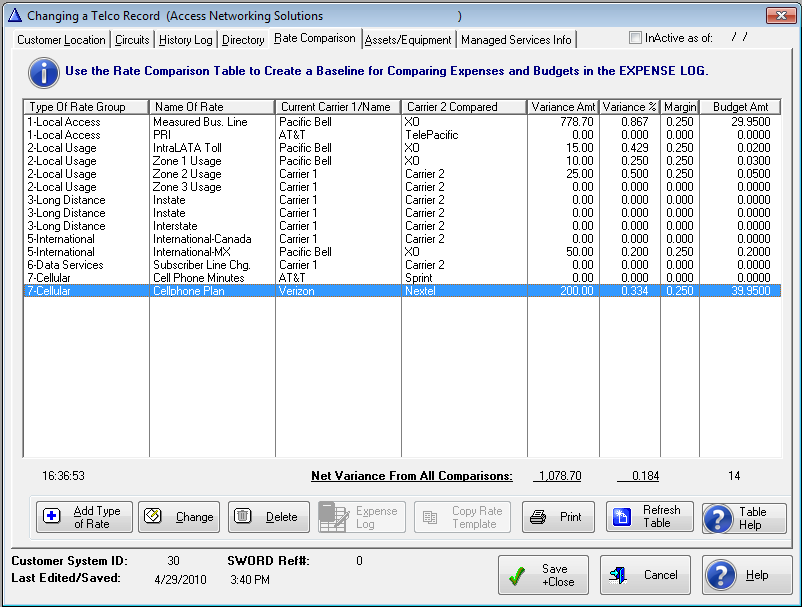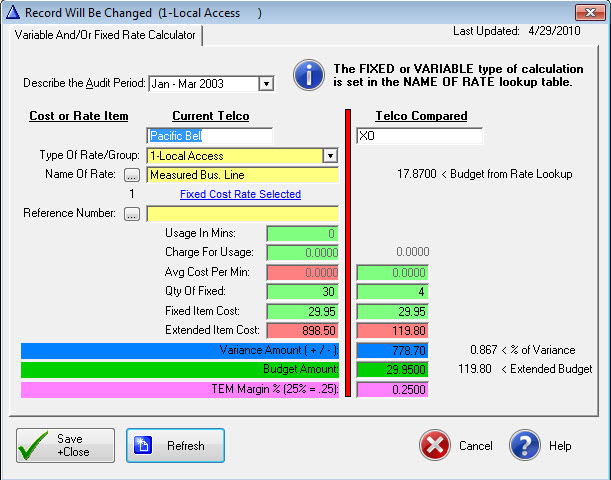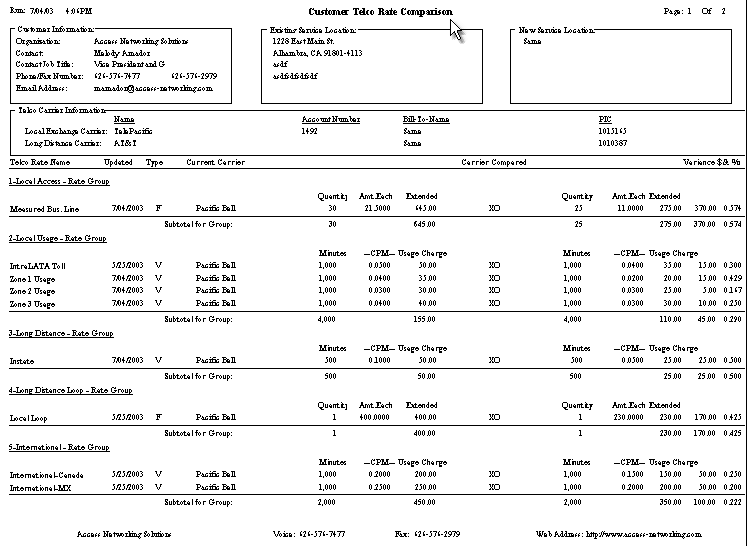﻿ Rate Comparisons | Telecommunications Expense Management Software

# TelcoMgr™ Rate Comparisons

## Tutorial

Select the [Rate Comparison] Tab.

A Rate Comparison can be constructed in this window or a pre-built Rate Comparison Template can be copied from the Rate Comparison Template library.To construct a Rate Comparison Table select the [Insert Button] a the Telco Rate Calculator or Rate Edit window will open. We recommend for users to create the Type of Rate (aka Rate Group Names) and the Name of Rate (aka Telco Rate/Zone Name, Rate Name) lookup tables before creating the first Rate Comparison for a Customer/location.Rate Comparison Edit Window handles Fixed or Variable Charges

Describe the [Audit Period] for the comparison.

Enter the [Current Telco] name and the name [Telco Compared].

In the [Type Of Rate] field enter '1-Local Access'. Alternately you may select from the drop-down box. The number 1 is entered before the Group Name to 'group' or list rate items together on the Rate Comparison report and the previous browse window.

In the [Name of Rate] field enter 'Measured Bus. Line' Enter '20' into the [Qty of Fixed] cost items. Enter \$20.00 in the [Fixed Item Cost] field.  The [Extended Item Cost] field is automatically calculated.

Use the [Refnumber] field to further identify a specific service. The [Select] button will open a window of the Lines, Features and Services associated with the Account.

The [Variance Amount ( +/ - )] is computed by the formula (Current Telco Extended Usage or Extended Item Amount - Telco Compared Extended Usage or Extended Item Amount).  A positive number represents a savings and negative (-) number represents an increase over the Current Amount.

The [Variance Percent ( + / - )] is computed by the formula (Variance Amount/Current Telco Extended Usage or Extended Item Amount.

The [Budget Amount] field should represent the quantity one cost of an entry; the cost of one minute if a variable cost or the cost of one item or service if a fixed, or recurring cost.

The Extended Budget (not shown) amount equals the Budget Amount multiplied times the quantity of fixed items or times the usage in minutes.

The [Margin] represents a percentage amount of the formula ((Extended Budget Amount - Extended Actual Amount) * Margin)). Margin amounts are computed in the Expense Log window and reported on the Expense Reports. The Margin can be used as an incentive for an individual, or team bonus, or commission for an independent consultant.

Tutorial Summary

Under the [Telco Compared] column enter \$21.50 into the [Fixed Item Cost] field. The [Extended Item Cost] field under the [Telco Compared] column is automatically calculated. The Variance Amount and the Variance Percent are automatically computed.  The formula for the Variance is the Current Extended Cost - Compared Extended Cost.  A positive number represents a savings to you and a negative number represents a cost increase.

Select [Save +Close] to save the record.

Select the [Insert] button again to add the next rate comparison. When the Rate Calculator window opens you will notice that the [Current Telco] and [Telco Compared] fields have been automatically entered.  These may be changed if necessary.

 1 From the drop-down list select '1-Local Usage'.
 2 Enter 'IntraLATA Toll' in the [Name of Rate] field.
 3 Enter '800' in the [Usage In Mins] field.
 4 Enter '40.00' in the [Charge For Usage] field.
 5 The [Avg. Cost Per Min] is automatically computed.
 6 In the [Telco Compared] column in the [Avg. Cost Per Min] field enter '.045'.  Essentially, this should be the amount that has been offered/quoted by the Telco that is being Compared.  Note that the [Charge For Usage] under the [Telco Compared] column is automatically computed.  The formula is the Current Telco [Usage in Mins] times the Compared Telco [Avg. Cost Per Min].
 7 The Variance Amount and Percent are automatically computed.

Repeat the process for each Type Of Rate and Name of Rate that you wish to compare.  A complete Rate Comparison Table is shown below:

To print your completed Rate Comparison Table select the [Print] option from the Main Menu.

A sample Telco Rate Comparison Report is shown below: